﻿ 带有变号权函数的p(x)-Kirchhoff类型方程正解的存在性与多重性

# 带有变号权函数的p(x)-Kirchhoff类型方程正解的存在性与多重性Multiplicity of Positive Solutions of p(x)-Kirchhoff Problems with Sign-Changing Weight Functions

Abstract: In this paper, the p(x)-Kirchhoff problem with sign-changing weight functions is studied. Based on variational method and Nehari manifold, it is proved the existence and multiplicity of positive so-lutions of the problem.

1. 引言

$\left\{\begin{array}{l}-M\left({\int }_{\Omega }\frac{1}{p\left(x\right)}{|\nabla u|}^{p\left(x\right)}\text{d}x\right){\Delta }_{p\left(x\right)}u=\lambda f\left(x\right){|u|}^{q\left(x\right)-2}u+g\left(x\right){|u|}^{h\left(x\right)-2}u,\text{ }\text{ }x\in \Omega ,\hfill \\ u=0,\text{ }\text{ }\text{ }\text{ }\text{ }\text{ }\text{ }\text{ }\text{ }\text{ }\text{ }\text{ }\text{ }\text{ }\text{ }\text{ }\text{ }\text{ }\text{ }\text{ }\text{ }\text{ }\text{ }\text{ }\text{\hspace{0.17em}}\text{\hspace{0.17em}}\text{ }\text{\hspace{0.17em}}\text{\hspace{0.17em}}\text{ }x\in \partial \Omega \hfill \end{array}$ (1)

(H1) 对于 $k\ge 0$$M\left(t\right)=a+b{t}^{k}$$t\in \left[0,\infty \right)$

(H2) $f\left(x\right)\in {L}^{{s}_{1}\left(x\right)}\left(\Omega \right)$$f\left(x\right)\overline{)\equiv }0$

(H3) $g\left(x\right)\in {L}^{{s}_{2}\left(x\right)}\left(\Omega \right)$$g\left(x\right)\overline{)\equiv }0$

(H4) $1，其中 $x\in \stackrel{¯}{\Omega }$

$1<{p}^{-}:=\underset{x\in \Omega }{\mathrm{inf}}p\left(x\right)\le p\left(x\right)\le {p}^{+}:=\underset{x\in \Omega }{\mathrm{sup}}p\left(x\right)$

$1<{q}^{-}\le {q}^{+}<{p}^{-}\le {p}^{+}<{p}^{+}\left(k+1\right)<{h}^{-}\le {h}^{+}$

2. 预备知识与变分框架

$p\left(x\right)\in {C}_{+}\left(\stackrel{¯}{\Omega }\right)$，变指数Lebesgue空间 ${L}^{p\left(\cdot \right)}\left(\Omega \right)$ 定义为：

${L}^{p\left(\cdot \right)}\left(\Omega \right)=\left\{u|u是一个可测实函数，\text{\hspace{0.17em}}{\int }_{\Omega }{|u|}^{p\left(x\right)}\text{d}x<\infty \right\},$

${|u|}_{p\left(x\right)}=\mathrm{inf}\left\{\lambda >0:{\int }_{\Omega }{|\frac{u\left(x\right)}{\lambda }|}^{p\left(x\right)}\text{d}x\le 1\right\}.$

${|u|}_{p\left(x\right)q\left(x\right)}\le 1⇒{|u|}_{p\left(x\right)q\left(x\right)}^{{p}^{+}}\le {|{|u|}^{p\left(x\right)}|}_{q\left(x\right)}\le {|u|}_{p\left(x\right)q\left(x\right)}^{{p}^{-}},$

${|u|}_{p\left(x\right)q\left(x\right)}\ge 1⇒{|u|}_{p\left(x\right)q\left(x\right)}^{{p}^{-}}\le {|{|u|}^{p\left(x\right)}|}_{q\left(x\right)}\le {|u|}_{p\left(x\right)q\left(x\right)}^{{p}^{+}}.$

${\int }_{\Omega }f\left(x\right){|u|}^{q\left(x\right)}\text{d}x\le 2{|f|}_{{s}_{1}\left(x\right)}{|{|u|}^{q\left(x\right)}|}_{{{s}^{\prime }}_{1}\left(x\right)}\le \mathrm{max}\left\{{C}_{1}{‖u‖}^{{q}^{+}},{C}_{2}{‖u‖}^{{q}^{-}}\right\},$ (2.1)

${\int }_{\Omega }g\left(x\right){|u|}^{h\left(x\right)}\text{d}x\le 2{|g|}_{{s}_{2}\left(x\right)}{|{|u|}^{h\left(x\right)}|}_{{{s}^{\prime }}_{2}\left(x\right)}\le \mathrm{max}\left\{{C}_{3}{‖u‖}^{{h}^{+}},{C}_{4}{‖u‖}^{{h}^{-}}\right\}.$ (2.2)

$\begin{array}{l}a{\int }_{\Omega }{|\nabla u|}^{p\left(x\right)-2}\nabla u\nabla \phi \text{d}x+b{\left({\int }_{\Omega }\frac{1}{p\left(x\right)}{|\nabla u|}^{p\left(x\right)}\text{d}x\right)}^{k}{\int }_{\Omega }{|\nabla u|}^{p\left(x\right)-2}\nabla u\nabla \phi \text{d}x\\ =\lambda {\int }_{\Omega }f\left(x\right){|u|}^{q\left(x\right)-2}u\phi \text{d}x+{\int }_{\Omega }g\left(x\right){|u|}^{h\left(x\right)-2}u\phi \text{d}x.\end{array}$

$\begin{array}{l}{J}_{\lambda }\left(u\right)=a{\int }_{\Omega }\frac{1}{p\left(x\right)}{|\nabla u|}^{p\left(x\right)}\text{d}x+\frac{b}{k+1}{\left({\int }_{\Omega }\frac{1}{p\left(x\right)}{|\nabla u|}^{p\left(x\right)}\text{d}x\right)}^{k+1}\\ \text{ }\text{ }\text{ }\text{ }\text{ }-\lambda {\int }_{\Omega }\frac{f\left(x\right)}{q\left(x\right)}{|u|}^{q\left(x\right)}\text{d}x-{\int }_{\Omega }\frac{g\left(x\right)}{h\left(x\right)}{|u|}^{h\left(x\right)}\text{d}x,\end{array}$ (2.3)

$‖u‖>1$ 时，有

$\begin{array}{l}{J}_{\lambda }\left(u\right)\ge \frac{a}{{p}^{+}}{\int }_{\Omega }{|\nabla u|}^{p\left(x\right)}\text{d}x+\frac{b}{{\left({p}^{+}\right)}^{k+1}\left(k+1\right)}{\left({\int }_{\Omega }{|\nabla u|}^{p\left(x\right)}\text{d}x\right)}^{k+1}\\ \text{ }\text{ }\text{ }\text{ }\text{ }-\frac{\lambda }{{q}^{-}}{\int }_{\Omega }f\left(x\right){|u|}^{q\left(x\right)}\text{d}x-\frac{1}{{h}^{-}}{\int }_{\Omega }g\left(x\right){|u|}^{h\left(x\right)}\text{d}x\\ \text{ }\text{ }\text{\hspace{0.17em}}\text{\hspace{0.17em}}\text{\hspace{0.17em}}\ge \frac{a}{{p}^{+}}{‖u‖}^{{p}^{-}}+\frac{b}{{\left({p}^{+}\right)}^{k+1}\left(k+1\right)}{‖u‖}^{{p}^{-}\left(k+1\right)}-\frac{\lambda }{{q}^{-}}{C}_{1}{‖u‖}^{{q}^{+}}-\frac{1}{{h}^{-}}{C}_{3}{‖u‖}^{{h}^{+}}.\end{array}$

${N}_{\lambda }=\left\{u\in E\\left\{0\right\}:〈{{J}^{\prime }}_{\lambda }\left(u\right),u〉=0\right\}.$

$\begin{array}{l}{I}_{\lambda }\left(u\right)=〈{{J}^{\prime }}_{\lambda }\left(u\right),u〉=a{\int }_{\Omega }{|\nabla u|}^{p\left(x\right)}\text{d}x+b{\left({\int }_{\Omega }\frac{1}{p\left(x\right)}{|\nabla u|}^{p\left(x\right)}\text{d}x\right)}^{k}{\int }_{\Omega }{|\nabla u|}^{p\left(x\right)}\text{d}x\\ \text{ }\text{ }\text{ }\text{ }\text{ }\text{ }\text{ }\text{ }\text{ }-\lambda {\int }_{\Omega }f\left(x\right){|u|}^{q\left(x\right)}\text{d}x-{\int }_{\Omega }g\left(x\right){|u|}^{h\left(x\right)}\text{d}x=0\end{array}$ (2.4)

$u\in {N}_{\lambda }$，有

$\begin{array}{l}〈{{I}^{\prime }}_{\lambda }\left(u\right),u〉=a{\int }_{\Omega }p\left(x\right){|\nabla u|}^{p\left(x\right)}\text{d}x+kb{\left({\int }_{\Omega }\frac{1}{p\left(x\right)}{|\nabla u|}^{p\left(x\right)}\text{d}x\right)}^{k-1}{\left({\int }_{\Omega }{|\nabla u|}^{p\left(x\right)}\text{d}x\right)}^{2}\\ \text{ }\text{ }\text{ }\text{ }\text{\hspace{0.17em}}\text{\hspace{0.17em}}\text{\hspace{0.17em}}+b{\left({\int }_{\Omega }\frac{1}{p\left(x\right)}{|\nabla u|}^{p\left(x\right)}\text{d}x\right)}^{k}{\int }_{\Omega }p\left(x\right){|\nabla u|}^{p\left(x\right)}\text{d}x-\lambda {\int }_{\Omega }f\left(x\right)q\left(x\right){|u|}^{q\left(x\right)}\text{d}x\\ \text{ }\text{ }\text{ }\text{ }\text{\hspace{0.17em}}\text{\hspace{0.17em}}\text{\hspace{0.17em}}-{\int }_{\Omega }g\left(x\right)h\left(x\right){|u|}^{h\left(x\right)}\text{d}x.\end{array}$ (2.5)

${N}_{\lambda }$ 分解为

${N}_{\lambda }^{+}\left(\Omega \right)=\left\{u\in {N}_{\lambda }\left(\Omega \right):〈{{I}^{\prime }}_{\lambda }\left(u\right),u〉>0\right\},$

${N}_{\lambda }^{-}\left(\Omega \right)=\left\{u\in {N}_{\lambda }\left(\Omega \right):〈{{I}^{\prime }}_{\lambda }\left(u\right),u〉<0\right\},$

${N}_{\lambda }^{0}\left(\Omega \right)=\left\{u\in {N}_{\lambda }\left(\Omega \right):〈{{I}^{\prime }}_{\lambda }\left(u\right),u〉=0\right\}.$

3. 预备引理

$\begin{array}{l}0=〈{{I}^{\prime }}_{\lambda }\left(u\right),u〉=a{{\int }_{\Omega }p\left(x\right){|\nabla u|}^{p\left(x\right)}\text{d}x+kb{\left({\int }_{\Omega }\frac{1}{p\left(x\right)}{|\nabla u|}^{p\left(x\right)}\text{d}x\right)}^{k-1}\left({\int }_{\Omega }{|\nabla u|}^{p\left(x\right)}\text{d}x\right)}^{2}\\ \text{ }\text{ }\text{ }\text{ }\text{\hspace{0.17em}}\text{\hspace{0.17em}}\text{\hspace{0.17em}}\text{ }\text{\hspace{0.17em}}\text{\hspace{0.17em}}+b{\left({\int }_{\Omega }\frac{1}{p\left(x\right)}{|\nabla u|}^{p\left(x\right)}\text{d}x\right)}^{k}{\int }_{\Omega }p\left(x\right){|\nabla u|}^{p\left(x\right)}\text{d}x-\lambda {\int }_{\Omega }f\left(x\right)q\left(x\right){|u|}^{q\left(x\right)}\text{d}x-{\int }_{\Omega }g\left(x\right)h\left(x\right){|u|}^{h\left(x\right)}\text{d}x\\ \text{ }\text{ }\text{ }\text{ }\text{ }\text{\hspace{0.17em}}\text{\hspace{0.17em}}\ge {p}^{-}a{\int }_{\Omega }{|\nabla u|}^{p\left(x\right)}\text{d}x+{p}^{-}\left(k+1\right)b{\left({\int }_{\Omega }\frac{1}{p\left(x\right)}{|\nabla u|}^{p\left(x\right)}\text{d}x\right)}^{k}{\int }_{\Omega }{|\nabla u|}^{p\left(x\right)}\text{d}x-{h}^{+}{\int }_{\Omega }g\left(x\right){|u|}^{h\left(x\right)}\text{d}x\\ \text{ }\text{ }\text{ }\text{ }\text{ }\text{ }\text{\hspace{0.17em}}-{q}^{+}\left[a{\int }_{\Omega }{|\nabla u|}^{p\left(x\right)}\text{d}x+b{\left({\int }_{\Omega }\frac{1}{p\left(x\right)}{|\nabla u|}^{p\left(x\right)}\text{d}x\right)}^{k}{\int }_{\Omega }{|\nabla u|}^{p\left(x\right)}\text{d}x-{\int }_{\Omega }g\left(x\right){|u|}^{h\left(x\right)}\text{d}x\right]\\ \text{ }\text{ }\text{ }\text{ }\text{\hspace{0.17em}}\text{\hspace{0.17em}}\text{\hspace{0.17em}}\text{\hspace{0.17em}}\text{\hspace{0.17em}}\text{\hspace{0.17em}}\ge \left({p}^{-}-{q}^{+}\right)a{‖u‖}^{{p}^{-}}+\frac{{p}^{-}\left(k+1\right)-{q}^{+}}{{\left({p}^{+}\right)}^{k}}b{‖u‖}^{{p}^{-}\left(k+1\right)}+\left({q}^{-}-{h}^{+}\right){C}_{3}{‖u‖}^{{h}^{+}}\end{array}$

$‖u‖\ge {C}_{5}{\left(\frac{{p}^{-}-{q}^{+}}{{h}^{+}-{q}^{+}}\right)}^{\frac{1}{{h}^{+}-{p}^{-}}}$ (3.1)

$\begin{array}{c}0=〈{{I}^{\prime }}_{\lambda }\left(u\right),u〉\\ \le {p}^{+}a{\int }_{\Omega }{|\nabla u|}^{p\left(x\right)}\text{d}x+\left(k+1\right){p}^{+}b{\left({\int }_{\Omega }\frac{1}{p\left(x\right)}{|\nabla u|}^{p\left(x\right)}\text{d}x\right)}^{k}{\int }_{\Omega }{|\nabla u|}^{p\left(x\right)}\text{d}x-\lambda {q}^{-}{\int }_{\Omega }f\left(x\right){|u|}^{q\left(x\right)}\text{d}x\\ \text{\hspace{0.17em}}\text{\hspace{0.17em}}-{h}^{-}\left[a{\int }_{\Omega }{|\nabla u|}^{p\left(x\right)}\text{d}x+b{\left({\int }_{\Omega }\frac{1}{p\left(x\right)}{|\nabla u|}^{p\left(x\right)}\text{d}x\right)}^{k}{\int }_{\Omega }{|\nabla u|}^{p\left(x\right)}\text{d}x-\lambda {\int }_{\Omega }f\left(x\right){|u|}^{q\left(x\right)}\text{d}x\right]\\ \le \left({p}^{\text{+}}-{h}^{-}\right)a{‖u‖}^{{p}^{-}}+\frac{{p}^{+}\left(k+1\right)-{h}^{-}}{{\left({p}^{-}\right)}^{k}}b{‖u‖}^{{p}^{-}\left(k+1\right)}+\lambda \left({h}^{-}-{q}^{-}\right){C}_{1}{‖u‖}^{{q}^{+}}\end{array}$

$‖u‖\le {C}_{6}{\left[\lambda \frac{{h}^{-}-{q}^{-}}{{h}^{-}-\left(k+1\right){p}^{+}}\right]}^{\frac{1}{{p}^{-}-{q}^{+}}}$ (3.2)

$\lambda$ 足够的小，例如

${\lambda }_{1}=\frac{{h}^{-}-{p}^{+}\left(k+1\right)}{{h}^{-}-{q}^{-}}{\left(\frac{1}{{C}_{6}}\right)}^{{p}^{-}-{q}^{+}}$

${\alpha }_{\lambda }^{+}=\underset{u\in {N}_{\lambda }^{+}\left(\Omega \right)}{\mathrm{inf}}{J}_{\lambda }\left(u\right)$${\alpha }_{\lambda }^{-}=\underset{u\in {N}_{\lambda }^{-}\left(\Omega \right)}{\mathrm{inf}}{J}_{\lambda }\left(u\right)$

$\begin{array}{l}{J}_{\lambda }\left(u\right)\ge \frac{a}{{p}^{+}}{\int }_{\Omega }{|\nabla u|}^{p\left(x\right)}\text{d}x+\frac{b}{{p}^{+}\left(k+1\right)}{\left({\int }_{\Omega }\frac{1}{p\left(x\right)}{|\nabla u|}^{p\left(x\right)}\text{d}x\right)}^{k}{\int }_{\Omega }{|\nabla u|}^{p\left(x\right)}\text{d}x-\frac{\lambda }{{q}^{-}}{\int }_{\Omega }f\left(x\right){|u|}^{q\left(x\right)}\text{d}x\\ \text{ }\text{ }\text{ }\text{ }\text{ }-\frac{1}{{h}^{-}}\left[a{\int }_{\Omega }{|\nabla u|}^{p\left(x\right)}\text{d}x+b{\left({\int }_{\Omega }\frac{1}{p\left(x\right)}{|\nabla u|}^{p\left(x\right)}\text{d}x\right)}^{k}{\int }_{\Omega }{|\nabla u|}^{p\left(x\right)}\text{d}x-\lambda {\int }_{\Omega }f\left(x\right){|u|}^{q\left(x\right)}\text{d}x\right]\\ \text{ }\text{ }\text{\hspace{0.17em}}\text{\hspace{0.17em}}\text{\hspace{0.17em}}\ge \left(\frac{a}{{p}^{+}}-\frac{a}{{h}^{-}}\right){‖u‖}^{{p}^{-}}+\left[\frac{1}{{p}^{+}\left(k+1\right)}-\frac{1}{{h}^{-}}\right]\frac{b}{{\left({p}^{+}\right)}^{k}}{‖u‖}^{{p}^{-}\left(k+1\right)}+{C}_{1}\lambda \left(\frac{1}{{h}^{-}}-\frac{1}{{q}^{-}}\right){‖u‖}^{{q}^{+}}\\ \text{ }\text{ }\text{\hspace{0.17em}}\text{\hspace{0.17em}}\ge \frac{{h}^{-}-{p}^{+}}{{p}^{+}{h}^{-}}a{‖u‖}^{{p}^{-}}+\frac{{h}^{-}-{p}^{+}\left(k+1\right)}{{\left({p}^{+}\right)}^{k+1}\left(k+1\right){h}^{-}}b{‖u‖}^{\left(k+1\right){p}^{-}}-{C}_{1}\lambda \frac{{h}^{-}-{q}^{-}}{{h}^{-}{q}^{-}}{‖u‖}^{{q}^{+}}\end{array}$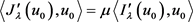(3.3)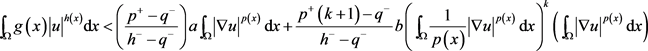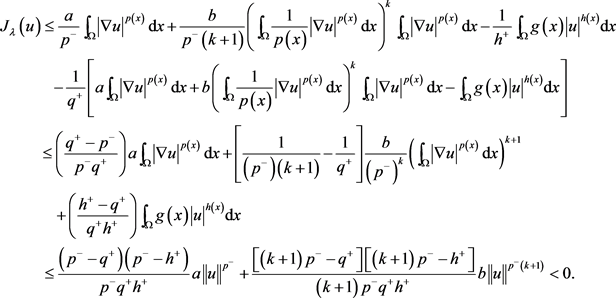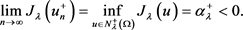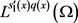中，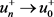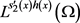中，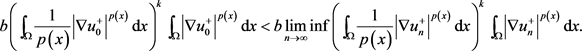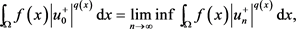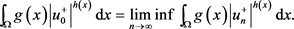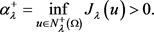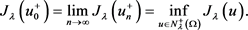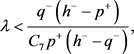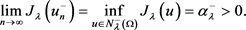中，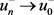中，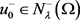，则存在常数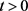使得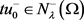以及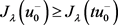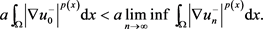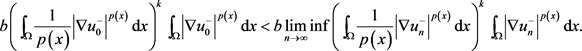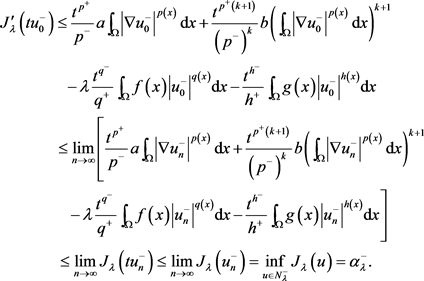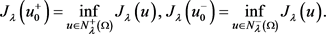Huang, J.C., Chen, C.S. and Xiu, Z.H. (2013) Existence and Multiplicity Results for a p-Kirchhoff Equation with a Concave-Convex Term. Applied Mathematics Letters, 26, 1070-1075.
https://doi.org/10.1016/j.aml.2013.06.001

 Chen, C.Y., Kuo, Y.C. and Wu, T.F. (2011) The Nehari Manifold for a Kirchhoff Type Problem Involving Sign- Changing Weight Functions. Journal of Differential Equations, 250, 1876-1908.
https://doi.org/10.1016/j.jde.2010.11.017

 Li, Y.X., Mei, M. and Zhang, K. (2016) Existence of Multiple Nontrivial Solutions for a p-Kirchhoff Type Elliptic Problem Involving Sign-Changing Weight Functions. Discrete and Continuous Dynamical Systems-Series B, 21, 883-908.
https://doi.org/10.3934/dcdsb.2016.21.883

 Khaled, B.A. (2012) Multiplicity of Positive Solution of p(x)-Laplacian Problems with Sign-Changing Weight Functions. Advances in Pure and Applied Mathematics, 3, 202-221.
https://doi.org/10.1515/apam-2012-0001

 Rasouli, S.H. and Fallah, K. (2017) The Nehari Manifold Approach for a p(x)-Laplacian Problem with Nonlinear Boundary Conditions. Ukrainian Mathematical Journal, 69, 111-125.
https://doi.org/10.1007/s11253-017-1350-6

 Fan, X.L., Shen, J.S. and Zhao, D. (2001) Sobolev Embedding Theorems for Spaces . Journal of Mathematical Analysis and Applications, 262, 749-760.
https://doi.org/10.1006/jmaa.2001.7618

 Zhang, X. and Liu, X. (2007) The Local Boundedness and Harnack Inequality of p(x)-Laplace Equation. Journal of Mathematical Analysis and Applications, 232, 209-218.

Top